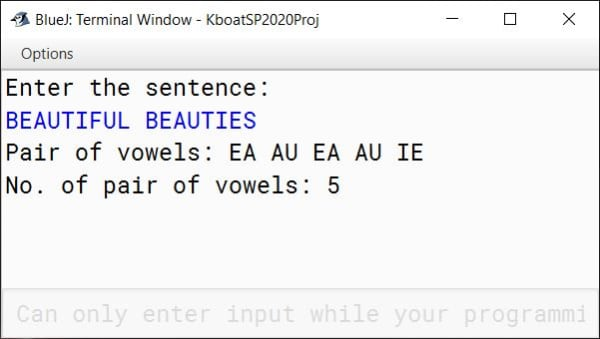# ICSE Computer Applications Specimen Paper 2020 Solution

## Section A

#### Question 1

(a) Define encapsulation.
The wrapping of data and methods that operate on that data into a single unit is called Encapsulation.

(b) Explain the purpose of using a 'new' keyword in a Java program.
The new keyword instantiates an array or a class by dynamically allocating memory for it at runtime and returning a reference to that memory.
For example,

`Employee emp = new Employee();`

Here we are creating an object of class Employee using the new keyword.

(c) What are literals?
Any constant value which can be assigned to the variable is called as literal. There are different types of literals like integer literals, floating point literals, character literals, String literals, boolean literals and null literals.

(d) Mention the types of access specifiers.
There are four types of access specifiers:

1. default
2. public
3. private
4. protected

Constructor overloading is a technique in Java through which a class can have more than one constructor with different parameter lists. The different constructors of the class are differentiated by the compiler using the number of parameters in the list and their types.
For example, a class Employee can have 3 constructors as shown below:

```Employee(long empId);
Employee(String name, double salary);
Employee(long empId, String name);```

Due to constructor overloading, all the 3 constructors are valid for Employee class.

#### Question 2

(a) Differentiate between boxing and unboxing.
Boxing is the conversion of primitive data type into an object of its corresponding wrapper class. Unboxing is the opposite of Boxing, it is the conversion of wrapper class object into its corresponding primitive data type.
Below program highlights the difference between the two:

```public class Boxing {
public static void main(String args[]) {
int a = 100, b;
//Boxing
Integer aWrapped = new Integer(a);
//Unboxing
b = aWrapped;
System.out.println("Boxed Value: " + aWrapped);
System.out.println("Unboxed Value: " + b);
}
}```

(b) Rewrite the following condition without using logical operators:

```if ( a>b || a>c )
System.out.println(a);```

Condition without using logical operators:

```if (a > b)
System.out.println(a);
else if (a > c)
System.out.println(a);```

(c) Rewrite the following loop using for loop:

```while (true)
System.out.print("*");```

Loop rewritten using for:

```for (;;)
System.out.print("*");```

(d) Write the prototype of a function search which takes two arguments a string and a character and returns an integer value.
Below is the function prototype required in the question:

`int search(String str, char ch);`

(e) Differentiate between = and == operators.

===
It is the assignment operator used for assigning a value to a variableIt is the equality operator used to check if a variable is equal to another variable or literal
E.g. int a = 10; assigns 10 to variable aE.g. if (a == 10) checks if variable a is equal to 10 or not

#### Question 3

(a) State the number of bytes and bits occupied by a character array of 10 elements. As size of char is 2 bytes, a character array of 10 elements occupies 2 x 10 = 20 bytes. 1 byte = 8 bits so 20 bytes convert to 8 x 20 = 160 bits.

(b) Differentiate between Binary Search and Linear Search techniques.

Linear SearchBinary Search
Linear search works on sorted and unsorted arraysBinary search works on only sorted arrays (ascending or descending)
Each element of the array is checked against the target value until the element is found or end of the array is reachedArray is successively divided into 2 halves and the target element is searched either in the first half or in the second half
Linear Search is slowerBinary Search is faster

(c) What is the output of the following:

```String a="Java is programming language \n developed by \t\'James Gosling\'";
System.out.println(a);```
##### Output
```Java is programming language
developed by    'James Gosling'
```

(d) Differentiate between break and System.exit(0).

breakSystem.exit(0)
break statement is used to jump out of the loop or switch-caseSystem.exit(0) terminates the entire program
break is a keywordSystem.exit(0) is a method provided by Java standard library

(e) Write a statement in Java for √((a+b)3÷|a-b|)
Math.sqrt(Math.pow(a + b, 3) / Math.abs(a - b));

(f) What is the value of m after evaluating the following expression:
m -= 9%++n + ++n/2; when int m=10,n=6
m = m - (9 % ++n + ++n / 2)
m = 10 - (9 % 7 + 8 / 2)
m = 10 - (2 + 4)
m = 10 - 6
m = 4

(g) Predict output of the following:
(i) Math.pow(25,0.5)+Math.ceil(4.2)

```10.0
```
##### Explanation

Math.pow(25,0.5) ⇒ √25 = 5.0
Math.ceil(4.2) = 5.0
5.0 + 5.0 = 10.0

(ii) Math.round ( 14.7 ) + Math.floor ( 7.9)

```22.0
```
##### Explanation

Math.round ( 14.7 ) = 15
Math.floor ( 7.9) = 7.0
15 + 7.0 = 22.0

(h) Give the output of the following java statements:
(i) "TRANSPARENT".toLowerCase();

##### Output
```transparent
```

(ii) "TRANSPARENT".compareTo("TRANSITION")

```7
```
##### Explanation

compareTo method will return the difference in ASCII codes of the first differing characters of both strings. The first differing characters of TRANSPARENT and TRANSITION are P and I, respectively at the 6th position of the strings. Output will be:
ASCII code of 'P' - ASCII code of 'I'
= 80 - 73
= 7

(i) Write a java statement for each to perform the following task:
(i) Find and display the position of the last space in a string str. System.out.println(str.lastIndexOf(' '));
(ii) Extract the second character of the string str.
System.out.println(str.charAt(1));

(j) State the type of errors if any in the following statements:
(i) switch ( n > 2 )
Syntax Error

##### Explanation

The expression of switch statement must resolve to byte, short, int, char or String. It cannot resolve to a boolean type so this statement will result in a syntax error.

(ii) System.out.println(100/0);
Runtime Error

##### Explanation

The program will compile successfully but when this line is executed it will result in "division by zero" error as dividing any number by 0 is an invalid division operation.

## Section B

#### Question 4

Anshul transport company charges for the parcels of its customers as per the following specifications given below:
Class name: Atransport
Member variables:
String name – to store the name of the customer
int w – to store the weight of the parcel in Kg
int charge – to store the charge of the parcel
Member functions:
void accept ( ) – to accept the name of the customer, weight of the parcel from the user (using Scanner class)
void calculate ( ) – to calculate the charge as per the weight of the parcel as per the following criteria:

Weight in KgCharge per Kg
Upto 10 KgsRs.25 per Kg
Next 20 KgsRs.20 per Kg
Above 30 KgsRs.10 per Kg

A surcharge of 5% is charged on the bill.
void print ( ) – to print the name of the customer, weight of the parcel, total bill inclusive of surcharge in a tabular form in the following format:
Name    Weight    Bill amount
-------    -------    -------------
Define a class with the above-mentioned specifications, create the main method, create an object and invoke the member methods.

```import java.util.Scanner;

public class Atransport
{
private String name;
private int w;
private int charge;

public void accept() {
Scanner in = new Scanner(System.in);
System.out.print("Enter Customer Name: ");
name = in.nextLine();
System.out.print("Enter Parcel Weight: ");
w = in.nextInt();
}

public void calculate() {
if (w <= 10)
charge = w * 25;
else if (w <= 30)
charge = 250 + ((w - 10) * 20);
else
charge = 250 + 400 + ((w - 30) * 10);

charge += charge * 5 / 100;
}

public void print() {
System.out.println("Name\tWeight\tBill amount");
System.out.println("----\t------\t-----------");
System.out.println(name + "\t" + w + "\t" + charge);
}

public static void main(String args[]) {
Atransport obj = new Atransport();
obj.accept();
obj.calculate();
obj.print();
}
}```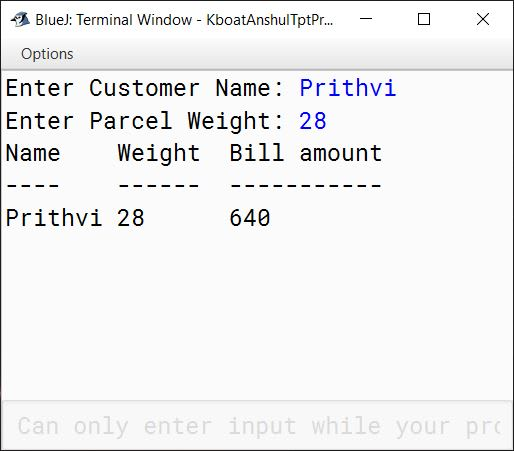#### Question 5

Write a program to input name and percentage of 35 students of class X in two separate one dimensional arrays. Arrange students details according to their percentage in the descending order using selection sort method. Display name and percentage of first ten toppers of the class.

```import java.util.Scanner;

public class KboatStudentPercentage
{
public static void main(String args[]) {
Scanner in = new Scanner(System.in);

//Initialize the 2 SDA
String names[] = new String;
double percentage[] = new double;

/*
* Accept student details from user and store
* in corresponding SDA
*/
for (int i = 0; i < names.length; i++) {
System.out.print("Enter name of student "
+ (i + 1) + ": ");
names[i] = in.nextLine();
System.out.print("Enter percentage of student "
+ (i + 1) + ": ");
percentage[i] = in.nextDouble();
in.nextLine();
}

//Selection Sort in Descending Order
for (int i = 0; i < percentage.length - 1; i++) {
int maxIdx = i;
for (int j = i + 1; j < percentage.length; j++) {
if (percentage[j] > percentage[maxIdx])
maxIdx = j;
}

double t = percentage[i];
percentage[i] = percentage[maxIdx];
percentage[maxIdx] = t;

String name = names[i];
names[i] = names[maxIdx];
names[maxIdx] = name;
}

//Display first ten toppers
System.out.println("Name\tPercentage");
for (int  i = 0; i < 10; i++) {
System.out.println(names[i] + '\t' + percentage[i]);
}
}
}```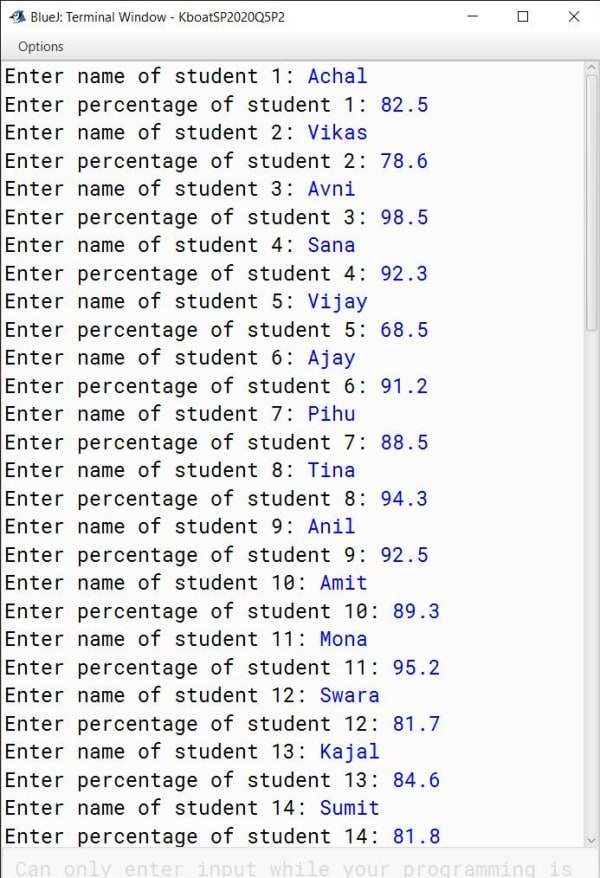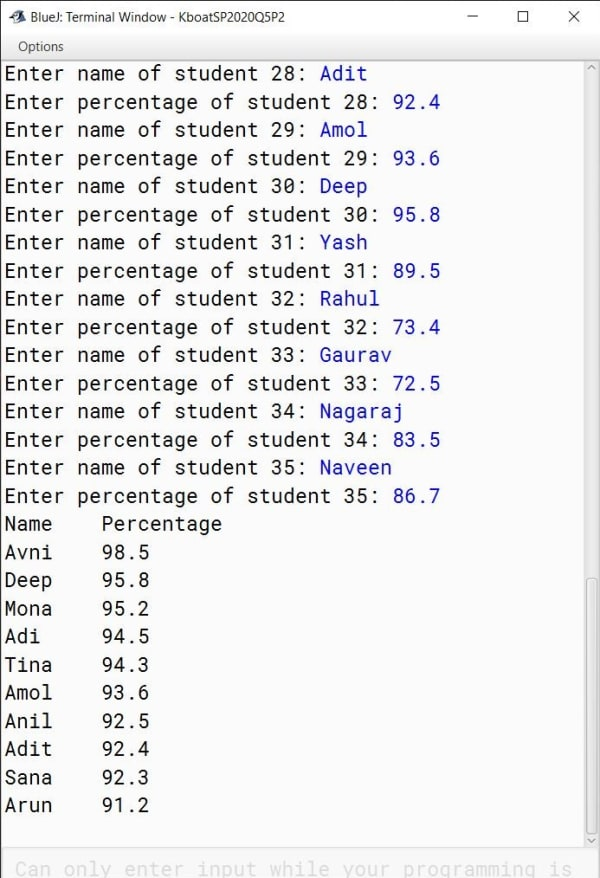#### Question 6

Design a class to overload a function Sum( ) as follows:
(i) int Sum(int A, int B) — with two integer arguments (A and B) calculate and return sum of all the even numbers in the range of A and B.
Sample input: A=4 and B=16
Sample output: sum = 4 + 6 + 8 + 10 + 12 + 14 + 16
(ii) double Sum( double N ) — with one double arguments(N) calculate and return the product of the following series:
sum = 1.0 x 1.2 x 1.4 x . . . . . . . . x N
(iii) int Sum(int N) - with one integer argument (N) calculate and return sum of only odd digits of the number N.
Sample input : N=43961
Sample output : sum = 3 + 9 + 1 = 13
Write the main method to create an object and invoke the above methods.

```public class KboatSumOverload
{
public int Sum(int A, int B) {
int sum = 0;
for (int i = A; i <= B; i++) {
if (i % 2 == 0)
sum += i;
}
return sum;
}

public double Sum(double N) {
double p = 1.0;
for (double i = 1.0; i <= N; i += 0.2)
p *= i;
return p;
}

public int Sum(int N) {
int sum = 0;
while (N != 0) {
int d = N % 10;
if (d % 2 != 0)
sum += d;
N /= 10;
}
return sum;
}

public static void main(String args[]) {

int evenSum = obj.Sum(4, 16);
System.out.println("Even sum from 4 to 16 = " + evenSum);

double seriesProduct = obj.Sum(2.0);
System.out.println("Series Product = " + seriesProduct);

int oddDigitSum = obj.Sum(43961);
System.out.println("Odd Digits Sum = " + oddDigitSum);
}
}```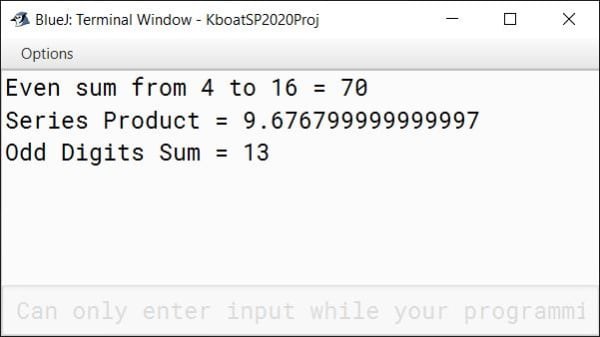#### Question 7

Using the switch statement, write a menu driven program to perform following operations:
(i) To Print the value of Z where Z = (x3 + 0.5x) / Y where x ranges from – 10 to 10 with an increment of 2 and Y remains constant at 5.5.
(ii) To print the Floyds triangle with N rows
Example: If N = 5, Output:
1
2 3
4 5 6
7 8 9 10
11 12 13 14

```import java.util.Scanner;

{
public static void main(String args[]) {
Scanner in = new Scanner(System.in);
System.out.println("Type 1 for Value of Z");
System.out.println("Type 2 for Floyd\'s triangle");

int choice = in.nextInt();

switch (choice) {
case 1:
double Z, Y = 5.5;
for (int x = -10; x <= 10; x+= 2) {
Z = (Math.pow(x, 3) + 0.5 * x) / Y;
System.out.println("Value of Z when x is "
+ x + " = " + Z);
}
break;

case 2:
System.out.print("Enter number of rows: ");
int N = in.nextInt();
int t = 1;
for (int i = 1; i <= N; i++) {
for (int j = 1; j <= i; j++) {
System.out.print(t + " ");
t++;
}
System.out.println();
}
break;

default:
System.out.println("Incorrect Choice");
break;
}
}
}```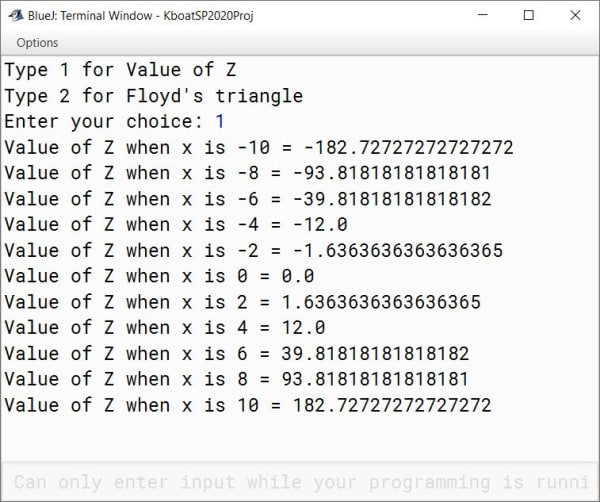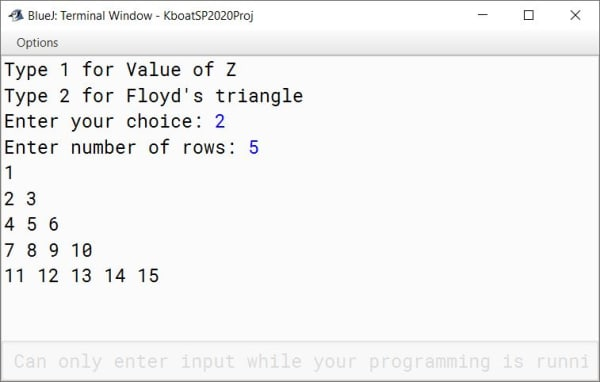#### Question 8

Write a program to input and store integer elements in a double dimensional array of size 4×4 and find the sum of all the elements.
7 3 4 5
5 4 6 1
6 9 4 2
3 2 7 5
Sum of all the elements: 73

```import java.util.Scanner;

public class KboatDDASum
{
public static void main(String args[]) {
Scanner in = new Scanner(System.in);

int arr[][] = new int;
long sum = 0;

System.out.println("Enter the elements of 4x4 array:");
for (int i = 0; i < 4; i++) {
for (int j = 0; j < 4; j++) {
arr[i][j] = in.nextInt();
}
}

for (int i = 0; i < 4; i++) {
for (int j = 0; j < 4; j++) {
sum += arr[i][j];
}
}

System.out.println("Sum of all the elements: " + sum);
}
}```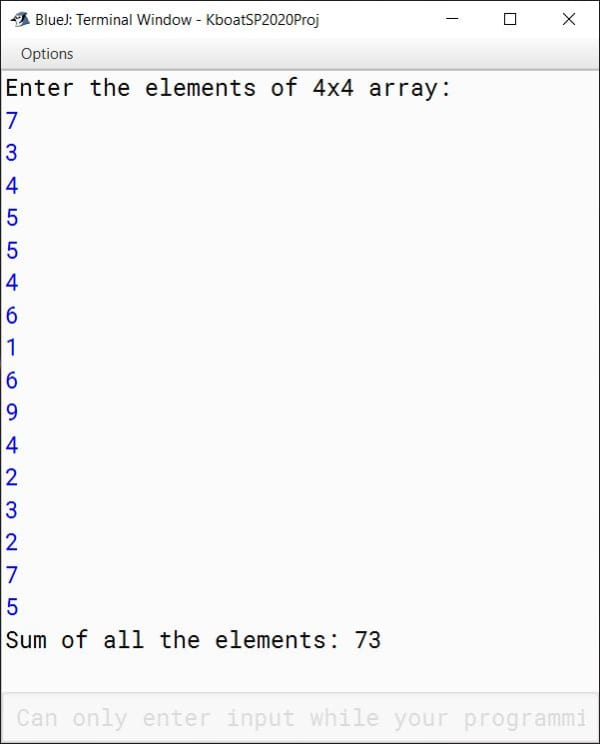#### Question 9

Write a program to input a string and convert it into uppercase and print the pair of vowels and number of pair of vowels occurring in the string.
Example:
Input:
"BEAUTIFUL BEAUTIES "
Output :
Pair of vowels: EA, AU, EA, AU, IE
No. of pair of vowels: 5

```import java.util.Scanner;

public class KboatVowelPair
{
static boolean isVowel(char ch) {
ch = Character.toUpperCase(ch);

if (ch == 'A' ||
ch == 'E' ||
ch == 'I' ||
ch == 'O' ||
ch == 'U')
return true;

return false;
}

public static void main(String args[]) {
Scanner in = new Scanner(System.in);
System.out.println("Enter the sentence:");
String str = in.nextLine();
str = str.toUpperCase();
int count = 0;

System.out.print("Pair of vowels: ");
for (int i = 0; i < str.length() - 1; i++) {
if (isVowel(str.charAt(i))
&& isVowel(str.charAt(i + 1))) {
count++;
System.out.print(str.charAt(i));
System.out.print(str.charAt(i+1) + " ");
}
}

System.out.print("\nNo. of pair of vowels: " + count);
}
}```